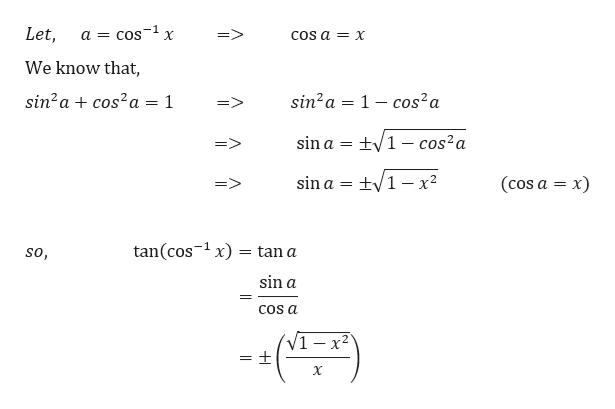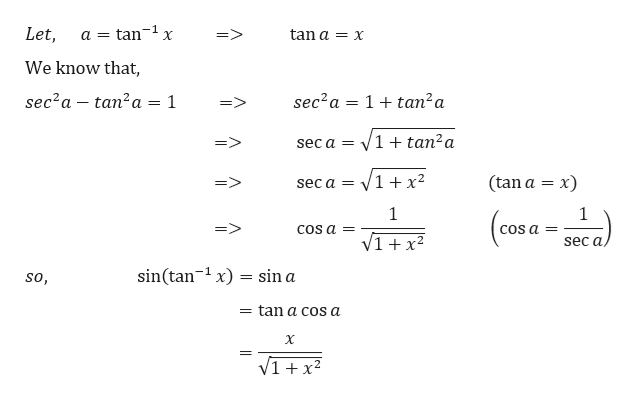# Simplify the expressions below.(a) tan(cos−1 x)  (b) sin(tan−1 x)

Question
6 views

Simplify the expressions below.

(a) tan(cos−1 x)

(b) sin(tan−1 x)
check_circle

Step 1

(a)help_outlineImage TranscriptioncloseLet, => COS a X a COS X We know that sin2acos2a 1 sin2a 1 -cos2a => tV1-cos2a sin a = (cos a x) sin a = tan(cos x) tan a so, sin a COs a _ = + X fullscreen
Step 2

Step 3

(b)

...help_outlineImage Transcriptionclose1 X Let, tan a= x => a tan We know that sec2a 1tan2a sec2a tan2a = 1 => V1 tan2a => sec a = (tan a x) V1+x2 sec a= 1 1 (cosa => COS a= V1x2 sec a sin(tan x)sin a -1 so, = tan a cos a X fullscreen

### Want to see the full answer?

See Solution

#### Want to see this answer and more?

Solutions are written by subject experts who are available 24/7. Questions are typically answered within 1 hour.*

See Solution
*Response times may vary by subject and question.
Tagged in

### Other